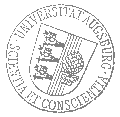Universität AugsburgInstitut für MathematikAugsburger Mathematisches Kolloquium

Dr. Matthias Wendt
Universität Wuppertal

spricht am

Dienstag, 18. Juni 2019

um

17:30 Uhr

im

Raum 2004 (L1)

über das Thema:

»Oriented Schubert calculus: using quadratic forms to count subspaces«

 Abstract: Classical Schubert calculus is a set of tools for computing degrees of intersections of Schubert varieties in Grassmannians. It can be used to answer enumerative geometry questions concerning intersections of subspaces of a vector space, at least over algebraically closed fields; the most classical such question is "How many lines in projective 3-space meet four given lines in general position?" Over general fields, like the real numbers, the answer is more complicated because some of the solution vector spaces may not be defined over the given field. To solve this issue, real enumerative geometry counts solutions with signs depending on additional orientations of the vector spaces to obtain refined information, such as lower bounds for the numbers of solutions. In the talk I will explain a refinement of the classical Schubert calculus which allows to compute the Chow-Witt rings of Grassmannians. In this refinement, the "number" of solution subspaces is a quadratic form which encodes both the classical answer (the number of solutions over the algebraic closure) as well as the signed counts from real enumerative geometry.

 Hierzu ergeht herzliche Einladung. Prof. Dr. Maxim Smirnov

Kaffee, Tee und Gebäck eine halbe Stunde vor Vortragsbeginn im Raum 2006 (L1).

[Impressum]      [Datenschutz]      wwwadm@math.uni-augsburg.de,     Do 11-Apr-2019 12:36:10 MESZ